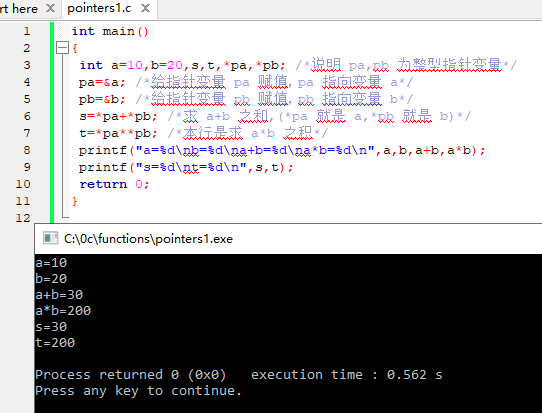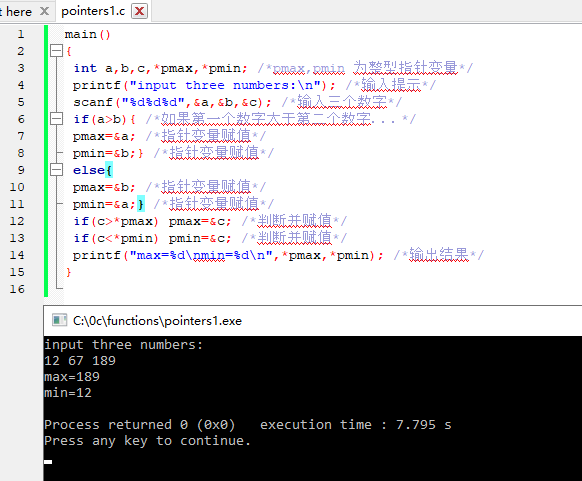# C语言 – 指针变量的运算

## 1. 赋值运算

A） 指针变量初始化赋值，前面已作介绍。
B）把一个变量的地址赋予指向相同数据类型的指针变量。

``````int a,*pa;
pa=&a; /*把整型变量 a 的地址赋予整型指针变量 pa*/``````

C） 把一个指针变量的值赋予指向相同类型变量的另一个指针变量。

``````int a,*pa=&a,*pb;
pb=pa; /*把 a 的地址赋予指针变量 pb*/``````

D） 把数组的首地址赋予指向数组的指针变量。

``````int a,*pa;
pa=a;``````

(数组名表示数组的首地址，故可赋予指向数组的指针变量 pa)也可写为：

``pa=&a; /* 数 组 第 一 个 元 素 的 地 址 也 是 整 个 数 组 的 首 地 址 ，也可赋予 pa*/``

``int a,*pa=a;``

E） 把字符串的首地址赋予指向字符类型的指针变量。

``````char *pc;
pc=”C Language”;``````

``char *pc=”C Language”;``

F） 把函数的入口地址赋予指向函数的指针变量。

``````int (*pf)();
pf=f; /*f 为函数名*/``````

## 2.指针运算符加减算术运算

``````int a,*pa;
pa=a; /*pa 指向数组 a，也是指向 a*/
pa=pa+2; /*pa 指向 a，即 pa 的值为&pa*/``````

## 3.两个指针变量之间的运算

A） 两指针变量相减：两指针变量相减所得之差是两个指针所指数组元素之间相差的元素个数。实际上是两个指针值(地址)相减之差再除以该数组元素的长度(字节数)。

B) 两指针变量进行关系运算：指向同一数组的两指针变量进行关系运算可表示它们所指数组元素之间的关系。

pf1==pf2 表示 pf1 和 pf2 指向同一数组元素；
pf1>pf2 表示 pf1 处于高地址位置；

pf1 指针变量还可以与 0 比较。

p!=0 表示 p 不是空指针。

``````#define NULL 0
int *p=NULL;``````

``````int main()
{
int a=10,b=20,s,t,*pa,*pb; /*说明 pa,pb 为整型指针变量*/
pa=&a; /*给指针变量 pa 赋值，pa 指向变量 a*/
pb=&b; /*给指针变量 pb 赋值，pb 指向变量 b*/
s=*pa+*pb; /*求 a+b 之和,(*pa 就是 a,*pb 就是 b)*/
t=*pa**pb; /*本行是求 a*b 之积*/
printf("a=%d\nb=%d\na+b=%d\na*b=%d\n",a,b,a+b,a*b);
printf("s=%d\nt=%d\n",s,t);
return 0;
}````````````main()
{
int a,b,c,*pmax,*pmin; /*pmax,pmin 为整型指针变量*/
printf("input three numbers:\n"); /*输入提示*/
scanf("%d%d%d",&a,&b,&c); /*输入三个数字*/
if(a>b){ /*如果第一个数字大于第二个数字．．．*/
pmax=&a; /*指针变量赋值*/
pmin=&b;} /*指针变量赋值*/
else{
pmax=&b; /*指针变量赋值*/
pmin=&a;} /*指针变量赋值*/

if(c>*pmax) pmax=&c; /*判断并赋值*/
if(c<*pmin) pmin=&c; /*判断并赋值*/
printf("max=%d\nmin=%d\n",*pmax,*pmin); /*输出结果*/
}``````Posted in C语言教程